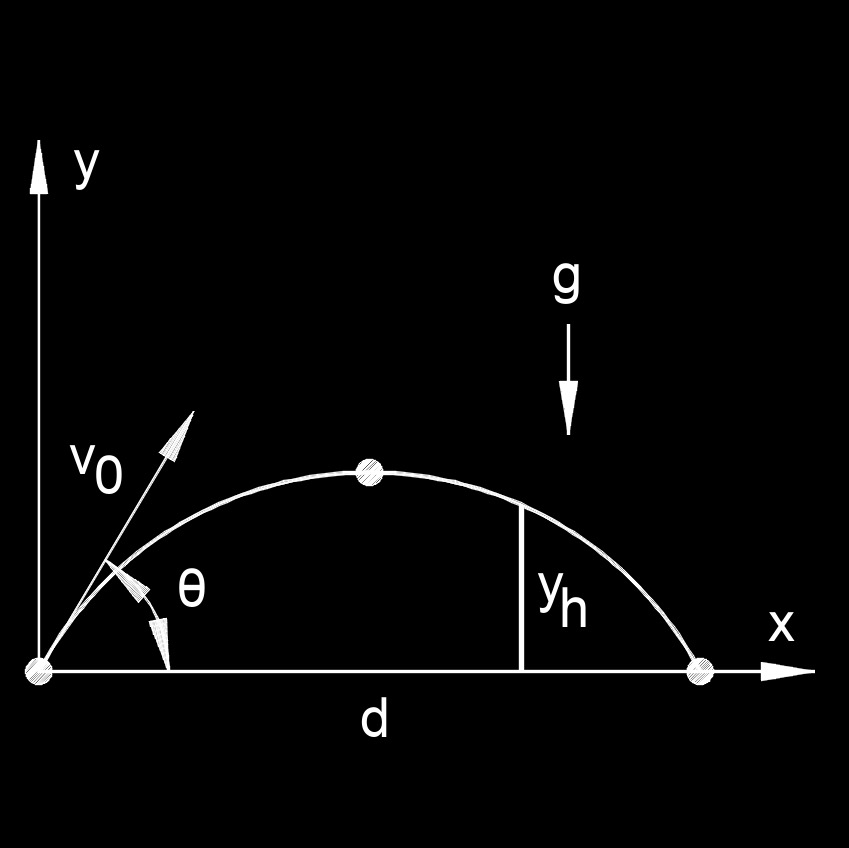# Trajectory of a Projectile

Written by Jerry Ratzlaff on . Posted in Classical Mechanics

Trajectory of a projectile is the curved path given an initial velocity and is acted on by gravity.  The projectile is acted upon by both vertical and horizontal motion along the trajectory.

##Trajectory of a Projectile formula

 $$\large{ y = d \; tan \; \theta - \frac{ g \; d^2 }{ 2 \; v_0^2 \; cos^2 \; \theta } }$$

### Where:

$$\large{ y }$$ = vertical position

$$\large{ \theta }$$ = angle of the initial vertical from x-axis

$$\large{ g }$$ = gravitational acceleration

$$\large{ d }$$ = horizontal position

$$\large{ v_0 }$$ = launch velocity

##Horizontal range of a Projectile formula

 $$\large{ R = \frac{ v_0^2 \; sin \; 2 \theta }{ g } }$$

### Where:

$$\large{ R }$$ = horizontal range

$$\large{ g }$$ = gravitational acceleration

$$\large{ v_0 }$$ = launch velocity

$$\large{ \theta }$$ = vertical angle

##Launch Velocity of a Projectile formula

 $$\large{ v_0 = \sqrt{ \frac{ R \; g }{ sin\; 2\theta } } }$$

### Where:

$$\large{ v_0 }$$ = launch velocity

$$\large{ R }$$ = horizontal range

$$\large{ g }$$ = gravitational acceleration

$$\large{ \theta }$$ = vertical angle

##Maximum Height of a Projectile formula

 $$\large{ h_{max} = \frac{ v_0 \; sin^2 \; \theta }{ 2 \; g } }$$

### Where:

$$\large{ h_{max} }$$ = maximum height

$$\large{ g }$$ = gravitational acceleration

$$\large{ v_0 }$$ = launch velocity

$$\large{ \theta }$$ = vertical angle

##Projectile Clearing an Object formula

 $$\large{ y_h = \frac{d \; v_{0y} }{v_{0x} } - \frac{1}{2} \; g \; \frac{x^2}{v_{0x}^2} }$$

### Where:

$$\large{ y_h }$$ = vertical position

$$\large{ \theta }$$ = angle of the initial vertical from x-axis

$$\large{ g }$$ = gravitational acceleration

$$\large{ d }$$ = horizontal position

$$\large{ v_0 }$$ = launch velocity

##Projectile Launch Angle formula

 $$\large{ \theta = \frac{ 1 }{ 2 } \; sin^{-1} \left( \frac{ g\; d }{ v_0^2 } \right) }$$

### Where:

$$\large{ \theta }$$ = angle of the initial vertical from x-axis

$$\large{ g }$$ = gravitational acceleration

$$\large{ d }$$ = horizontal position

$$\large{ v_0 }$$ = launch velocity

##Time of flight of a Projectile formula

 $$\large{ t = \frac{ 2 \; v_0 \; sin \; \theta }{ g } }$$

### Where:

$$\large{ t }$$ = time

$$\large{ g }$$ = gravitational acceleration

$$\large{ v_0 }$$ = launch velocity

$$\large{ \theta }$$ = vertical angle

##Trajectory of a Projectile on a Hill formulas

 $$\large{ d = v_{0d} \; t }$$ $$\large{ h = v_{0h} \; t - \frac{1}{2} \; g \; t^2 }$$ $$\large{ t = \frac{ 2 \; v_{0h} }{ g } \pm \sqrt{ \frac{ v_{0h}^2 }{ g^2 } - \frac{ 2 \;h }{ g } } }$$

### Where:

$$\large{ t }$$ = time

$$\large{ g }$$ = gravitational acceleration

$$\large{ v_0 }$$ = launch velocity

$$\large{ \theta }$$ = vertical angle